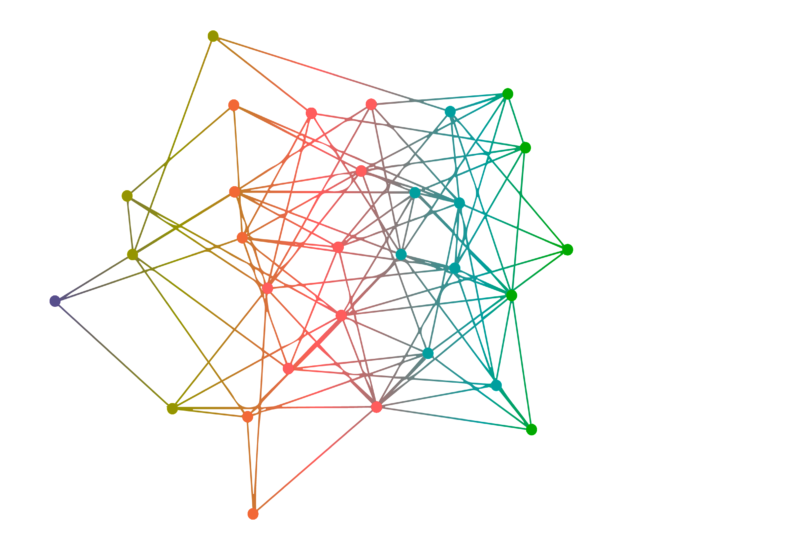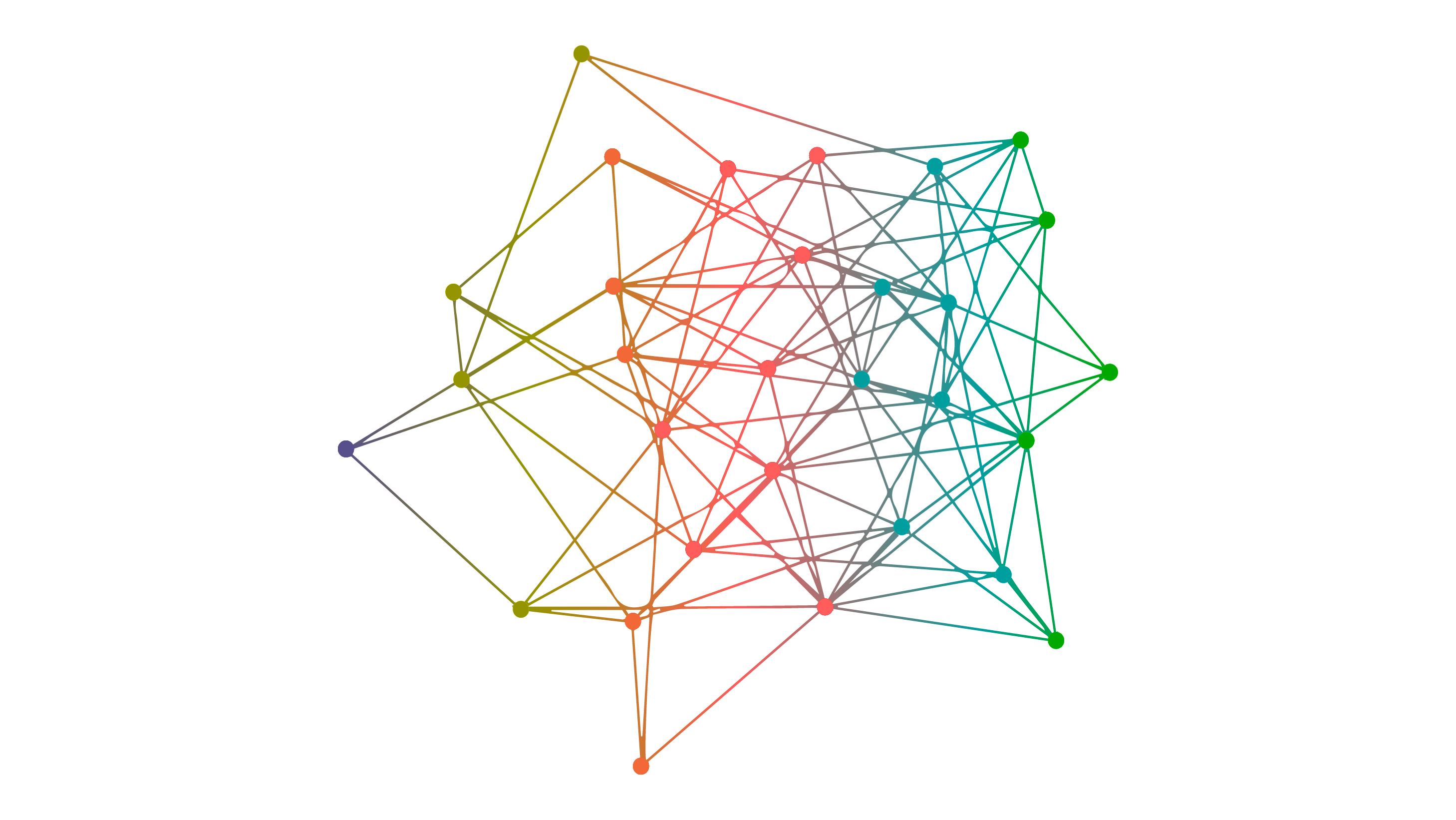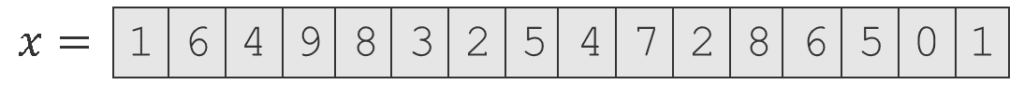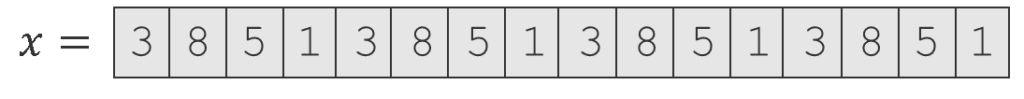# Microsoft Research Blog

The Microsoft Research blog provides in-depth views and perspectives from our researchers, scientists and engineers, plus information about noteworthy events and conferences, scholarships, and fellowships designed for academic and scientific communities.

## Quantum speedups for unstructured problems: Solving two twenty-year-old problems

May 4, 2020 | By Robin Kothari, Senior ResearcherOne of the goals of quantum computing research is to understand which problems quantum computers can solve faster than classical (non-quantum) computers and how big the speedup can be. Grover’s algorithm and Shor’s algorithm are two famous quantum algorithms that yield a polynomial speedup and an exponential speedup, respectively, over their classical counterparts.

In this context, a polynomial speedup is when a quantum computer solves a problem in time T, but a classical computer needs time T 2 (for example) or some other polynomial function of T. For example, Grover’s algorithm gives a quadratic (T versus T 2) speedup, which means it can solve a problem on a quantum computer with 1,000 steps that would take 1,000,000 steps on a classical computer. On the other hand, an exponential speedup is where a quantum computer takes time T but a classical computer takes time 2T (for example) or an exponentially growing function of T. Here, if T is 100, this is the difference between 100 and 2100. Such impressive speedups are one of the most promising and compelling aspects of quantum computers.

While we ultimately want speedups when executing on a real quantum device, we can study speedups theoretically and prove these speedups exist mathematically. To do so, we often study the problem in an abstract model of computation known as the “black-box model,” the query model, or the decision tree model. Most known quantum algorithms are either naturally stated in the query model or have a query algorithm at their core that is the source of the speedup. This includes algorithms like Grover’s algorithm, Shor’s algorithm, Simon’s algorithm, the Deutsch–Jozsa algorithm, the Bernstein–Vazirani algorithm, the quantum linear systems algorithm, quantum algorithms for solving semidefinite programs, and quantum algorithms for simulating sparse Hamiltonian dynamics.

My collaborators, Scott Aaronson from the University of Texas at Austin, Shalev Ben-David from the University of Waterloo, and Avishay Tal from the University of California, Berkeley, and I recently revisited the question of the largest possible quantum speedups for some important classes of problems. Specifically, we investigated the implications of a 2019 breakthrough by Hao Huang, which resolved a major open problem in the analysis of Boolean functions, called sensitivity conjecture. We found that his result proves a stronger claim with several implications for quantum query complexity. First, we showed that the best possible quantum speedup for unstructured problems is quartic (T versus T4 , described further below). This resolves a question that’s been open for over 20 years. Second, we found that the proof technique can also be used to resolve an old conjecture about quantum speedups for graph problems. These results are described in our paper, “Quantum Implications of Huang’s Sensitivity Theorem,” which can be found here.

Additional context for what this breakthrough means in the quantum computing world can be found in this accompanying blog post here

### Studying quantum speedups in the query model

In the query model, we have an input string x = (x1 , x2 , … , xn ) and our goal is to compute some function ƒ(x). However, the input x is not directly available to us—we must query a black box that knows x with an index i, and the black box will respond with the value of xi . The goal is to compute ƒ(x) while minimizing the number of queries to the black box. The only resource we minimize is the number of queries—not the time or memory used by the algorithm. The minimum number of queries needed to solve a problem is called its query complexity. For formal definitions and a survey of the query model, see this 2002 survey by Buhrman and de Wolf.For example, let x be a list of n numbers, and say we want to decide whether this list contains a given number, say the number 7. The obvious classical algorithm would query each xi and check if it equals this number. It can be shown that any classical algorithm that solves this problem must make $$\Theta$$(n) queries. However, a quantum algorithm that can make quantum queries in superposition to the black box can solve the problem with only $$\Theta$$$$(\sqrt[]{n})$$ queries using Grover’s algorithm. This shows a quadratic (or power 2) quantum speedup over classical algorithms.

As another example, in the element distinctness problem we have a list of numbers and want to decide whether all the numbers are distinct. While classical algorithms require $$\Theta$$(n) queries to solve this problem, Ambainis’ quantum algorithm only uses $$\Theta$$(n2/3) queries. In this case, quantum computers offer a power 1.5 speedup.On the other hand, there are problems with more dramatic speedups. Consider the period-finding problem that lies at the heart of Shor’s algorithm. Here, the input x is a list of n numbers promised to be periodic, which means it consists of a sequence of k distinct numbers that repeat over and over, such as x = (3,8,5,1,3,8,5,1,3,8,5,1). This string is periodic with period k = 4. The goal of the period-finding problem is to determine the period. Here, quantum algorithms provide an exponential speedup over classical algorithms.

Why do some problems allow exponential speedup, whereas others only allow polynomial speedup? The answer lies in the structure of the input. For the first two problems, where quantum computers only achieve polynomial speedup, we did not assume anything about the input. The input could be any list of n numbers. On the other hand, we assumed that the input was very special in the period-finding problem: it was a sequence of distinct numbers that repeated over and over. We call the first kind of problems unstructured problems or total functions.

### Speedups for Unstructured Problems

The relation between structure and speedup was recognized in a 1998 paper by Beals et al. If we use D(ƒ) and Q(ƒ) to denote the classical (deterministic) and quantum query complexity of a function ƒ, they showed that for all total functions ƒ: {0,1}n → {0,1}, we must have D(ƒ) = Ο(Q(ƒ)6). This means the maximum possible quantum speedup for an unstructured problem is power 6. Although this put an upper bound on the largest speedup possible, the largest speedup known at the time was only power 2, exhibited by Grover’s algorithm.

After more than 15 years, Ambainis et al. proved that a power 4 speedup was possible, showing that the maximum quantum speedup lies somewhere between power 4 and power 6. But what is the true answer?

Our work resolves this problem and shows that for all total functions ƒ, we have D(ƒ) = Ο(Q(ƒ)4).

So why do we care about the largest speedup for unstructured problems, when clearly the structured problems are the ones with greater speedups? First, structured problems rely on inputs having very special structure, which makes them less broadly applicable. For example, the task of searching a list for a specific item is a common subproblem in many situations, but finding out the period of a periodic string is not. Second, results like this inform our search for exponential speedups, so we know where to look for exponential speedups. When faced with a new problem, we can already rule out speedups larger than power 4 if the problem is unstructured.

### Speedups for Graph Problems

Graph problems are common source of algorithmic problems in computer science. A graph is defined by a set of vertices and a set of edges between vertices. For example, the vertices might correspond to people, and there is an edge between two people if they are friends. Now we might want to determine properties of the graph, such as if the graph is connected (Is there is a path of edges in the graph between any two people?), if the diameter of the graph is at most 6 (Are any two people connected by at most six degrees of separation?), or if the graph has a clique of size k (Is there a group of k people who are all friends with each other?).

In the query model, let’s assume the input is a graph specified by its adjacency matrix. This means we can query the black box with a pair of vertices u and v, and it will tell us whether there is an edge between u and v. Most common graph properties (including the examples above) are monotone, which means that if the graph satisfies the property, then adding more edges cannot destroy the property.

The query complexity of monotone graph properties has been studied since the 1970s. The Aanderaa–Karp–Rosenberg conjecture, which remains unsolved to this day, states that any classical randomized algorithm must make Ω(n2) queries to decide a monotone graph property.

The quantum analogue of this conjecture was first studied in 1999 by Buhrman et al. who showed that any quantum algorithm must make Ω$$(\sqrt[]{n})$$ queries to decide a monotone graph property. The conjectured answer was Ω(n), the best one could hope for as there are graph properties that can be solved with Ο(n)‏ quantum queries using Grover’s algorithm.

With the same proof technique, we also resolve this conjecture optimally. This is a surprising state of affairs as we are able to completely resolve the quantum analog of the conjecture while the classical version remains unsolved.

### Proof idea and outlook

Both the results presented above follow from our main technical result: for any total function ƒ, the quantum query complexity of ƒ is at least the square root of the polynomial degree of ƒ. The polynomial degree of ƒ is the least degree of any real polynomial p(x1, x2, … xn) that equals ƒ at all x∈ {0,1}n. We show this using the proof technique developed by Huang in a recent breakthrough solving a long-standing conjecture in the query model known as the sensitivity conjecture. Combining this main result with known relations yields the two results.

Now that we understand the maximum quantum speedup for unstructured problems, one tantalizing task is to find more examples of such problems. Ambainis et al. already exhibited one example of such a problem, but unfortunately their example is unlikely to be a problem that arises naturally. Can we next find natural examples of unstructured problems with larger quantum speedup than that offered by Grover’s algorithm?

## Up Next

Quantum computing, Security, privacy, and cryptography

### Post-quantum cryptography: Supersingular isogenies for beginners webinar

May 28, 2020 10:00 AM (PT) - In this webinar led by Microsoft researcher Dr. Craig Costello, you will examine why post-quantum cryptography is so critical as we move closer to realizing quantum computing, and you will learn the basics of supersingular isogeny Diffie-Hellman (SIDH), which is one of the popular candidates for post-quantum key exchange. The best known classical and quantum algorithms for attacking the SIDH protocol have exponential runtimes, which is why SIDH has the lowest bandwidth requirements of all the post-quantum encryption candidates currently under scrutiny.Microsoft blog editor

Quantum computing

### Harnessing the problem-solving power of quantum computing webinar

May 7, 2020 8:00 AM (PT) - In this webinar, Anita Ramanan, a Senior Quantum Software Engineer at Microsoft, will take you on a quantum journey, starting with a walk-through of potential applications and continuing with an explanation of what makes quantum computers so special. She’ll introduce the concept of the quantum bit, or qubit—the fundamental unit of quantum information—as well as the topological qubit, the novel implementation in development at Microsoft. She’ll also introduce Azure Quantum, which brings together all of the company’s quantum efforts, including hardware, cryo-control, the open-source Quantum Development Kit, and quantum-inspired optimization, which today can provide speedup using classical hardware and code by emulating certain quantum processes.Microsoft blog editor

Algorithms, Quantum computing, Security, privacy, and cryptography

### News from the front in the post-quantum crypto wars with Dr. Craig Costello

Episode 94, October 16, 2019 - Dr. Craig Costello is in the business of safeguarding your secrets. And he uses math to do it. A researcher in the Security and Cryptography group at Microsoft Research, Dr. Costello is among a formidable group of code makers (aka cryptographers) who make it their life’s work to protect the internet against adversarial code breakers (aka cryptanalysts), both those that exist today in our classical computing world, and those that will exist in a quantum computing future. On today’s podcast, Dr. Costello gives us a battlefield update in the ongoing crypto wars; talks about different approaches to post quantum cryptography and explains why he believes isogeny-based primitives are among the most promising; and reassures us that, as long as the battle goes on, cryptographers will continue to work very hard on the very hard math they hope will protect us from hackers and attackers, even in the age of quantum computers.Microsoft blog editor